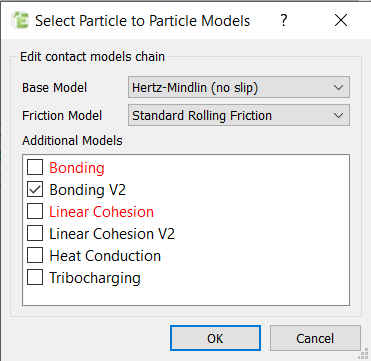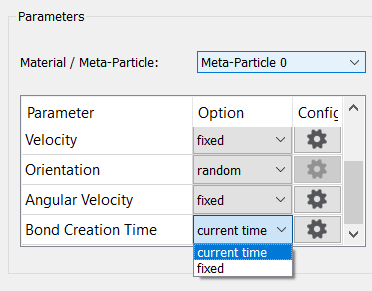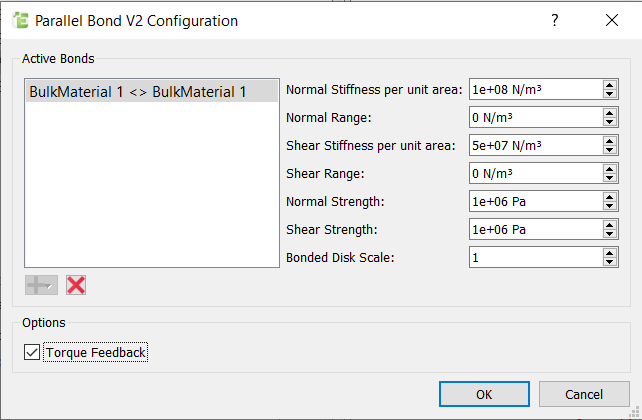# Bonding V2

The bonding V2 can be accessed via the Creator Physics tab. It is an updated GPU compatible model based on the original Bonding physics model.Bonds are created based on 2 methods, either ‘current time’ or ‘fixed’.  Once the bond model V2 is selected these options are shown in the Factory creating the Bulk Material or Meta-Particles. “Current time” allows the dynamic creation of bonded materials and “fixed” allows the creation of bonded material at one user defined point in time.If the Bulk Material Active Bonds are defined and the Particles that comprise of the Bulk Material are in contact at the Bond Creation Time then the bonds are formed according the bond parameters.  This occurs once per simulation for each particle pair. Once the bond time has passed no new bonds will be formed.The user also has the option to use a custom API factory to create material and use the Bonding V2 model.  The API factory can be set to assign a Custom Property with name CREATIONTIME = "Bond Creation Time”.  The factory must then assign a value to the custom property equal to the current simulation time. EDEM will then create bonds between particles if the particles are in contact and both particles have a Custom Property CREATIONTIME value equal to the current simulation time.  The custom property must be defined with 1 element and unit of eTime.

After bonding, the forces (Fn,t)/torques (Tn,t) on the particle are set to zero and are adjusted incrementally every time step according to:where:RB is the radius of the “glue”. This value is set as the radius of the smallest particle out of a contact pair multiplied by the Bonded Disk Scale value set by the user.

Sn,t are the normal and shear stiffness respectively. If the Normal or Tangential Range value is set to 0 N/m3 then the stiffness value is assigned based on the bond configuration value.  Otherwise the bond is assigned a value randomly distributed between the Stiffness value + or – the range value. This provides a linear distribution of bond stiffness values for a given material.

δt is the time step. vn,t are the normal and tangential velocities of the particles and ωn,t the normal and tangential angular velocities.

The bond is broken when the normal and tangential shear stresses exceed some predefined value:These bond forces/torques are in addition to the Base Contact Model. Since the bonds involved in this model can act when the particles are no longer physically in contact, the contact radius should be set to higher than the actual radius of the spheres. This model may only be used between particles.

 Interaction Configurable Parameters Position Particle to Particle Select an active bond then set the Bond Formation method and the following parameters:   Normal Stiffness per unit area: the tensile/compressive stiffness along the bond’s principal axis.   Normal Range: The applied Normal Stiffness per unit area value assigned to the bond pair is a value randomly. distributed between the Normal Stiffness value + or – the Normal range value.   Shear Stiffness per unit area: Shear stiffness in the orthogonal plane to the bond’s principal axis. Shear Range: The applied Shear Stiffness per unit area value assigned to the bond pair is a value randomly distributed between the Shear Stiffness value + or – the Shear range value.   Critical Normal Stress: the maximum normal stress the bond can withstand before it fails.   Critical Shear Stress: The maximum tangential stress the bond can withstand before it fails.   Bonded Disk Scale: The radius of the cylindrical bond between the particles will be equal to the smallest particle radius in a bond pair multiplied by the bonded disk scale value.   Torque Feedback: Enables the Bond Torque to be returned to the Particle.   Set the bond stress and stiffness values to represent the material that is been modeled. A high stiffness value will produce high bond forces and stresses. A lower than normal time step may be required to accurately capture these high forces.   When the Bond Formation Time is reached, all defined particles in contact will be bonded together. Before this time, particles interact based on the Hertz-Mindlin contact model.   Note that a bond between two particles will cease to exist whenever one or both of the bonded particles leaves the simulation domain. If periodic boundaries are applied when the particle leaves the domain, then the particle will exist on the opposite side of the domain; in this case the two particles will remain bonded across the periodic boundary.   When Torque Feedback is selected the bonded structure has a greater resistance to bending when considering a bond between two spheres, for a bonded structure where rotation is limited this setting has less impact. Last

At this time it is not possible to have the Sphero-Cylinder ends overlapping physically and there must be a small gap between each Sphero-Cylinder in the bonded structure.